Updating search results...

# 33 Results

View
Selected filters:
• MCCRS.Math.Content.6.G.A.1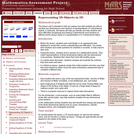Only Sharing Permitted
CC BY-NC-ND
Rating
0.0 stars

This lesson unit is intended to help teachers assess how well students are able to visualize two-dimensional cross-sections of representations of three-dimensional objects. In particular, the lesson will help you identify and help students who have difficulties recognizing and drawing two-dimensional cross-sections at different points along a plane of a representation of a three-dimensional object.

Subject:
Geometry
Mathematics
Material Type:
Assessment
Lesson Plan
Provider:
Shell Center for Mathematical Education
Provider Set:
Mathematics Assessment Project (MAP)
04/26/2013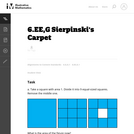Unrestricted Use
CC BY
Rating
0.0 stars

This is a task from the Illustrative Mathematics website that is one part of a complete illustration of the standard to which it is aligned. Each task has at least one solution and some commentary that addresses important asects of the task and its potential use. Here are the first few lines of the commentary for this task: Take a square with area 1. Divide it into 9 equal-sized squares. Remove the middle one. What is the area of the figure now? Take the remaining 8 square...

Subject:
Mathematics
Material Type:
Activity/Lab
Provider:
Illustrative Mathematics
Provider Set:
Illustrative Mathematics
Author:
Illustrative Mathematics
08/06/2013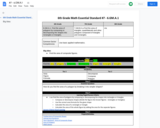Conditional Remix & Share Permitted
CC BY-NC-SA
Rating
0.0 stars

Find the area of polygons by composing or decomposing the shapes into rectangles or triangles.

Subject:
Mathematics
Material Type:
Activity/Lab
Author:
Liberty Public Schools
04/12/2021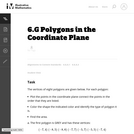Unrestricted Use
CC BY
Rating
0.0 stars

This is a task from the Illustrative Mathematics website that is one part of a complete illustration of the standard to which it is aligned. Each task has at least one solution and some commentary that addresses important asects of the task and its potential use. Here are the first few lines of the commentary for this task: The vertices of eight polygons are given below. For each polygon: * Plot the points in the coordinate plane connect the points in the order that they a...

Subject:
Mathematics
Material Type:
Activity/Lab
Provider:
Illustrative Mathematics
Provider Set:
Illustrative Mathematics
Author:
Illustrative Mathematics
09/08/2013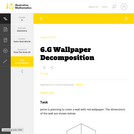Unrestricted Use
CC BY
Rating
0.0 stars

This is a task from the Illustrative Mathematics website that is one part of a complete illustration of the standard to which it is aligned. Each task has at least one solution and some commentary that addresses important aspects of the task and its potential use.

Subject:
Geometry
Mathematics
Material Type:
Activity/Lab
Provider:
Illustrative Mathematics
Provider Set:
Illustrative Mathematics
Author:
Illustrative Mathematics
08/06/2015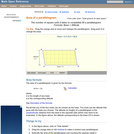Rating
0.0 stars

A web page and interactive applet showing the ways to calculate the area of a parallelogram. The user can drag the vertices of the parallelogram and the other points change automatically to ensure it remains a parallelogram. A grid inside the shape allows students to estimate the area visually, then check against the actual computed area, which is continuously recomputed and displayed. The text on the page gives three different ways to calculate the area with a formula for each. The applet uses one of the methods to compute the area in real time, so it changes as the rhombus is reshaped with the mouse. A companion page is http://www.mathopenref.com/parallelogram.html showing the definition and properties of a parallelogram Applet can be enlarged to full screen size for use with a classroom projector. This resource is a component of the Math Open Reference Interactive Geometry textbook project at http://www.mathopenref.com.

Subject:
Geometry
Mathematics
Material Type:
Simulation
Provider:
Math Open Reference
Author:
John Page
02/16/2011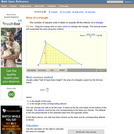Rating
0.0 stars

An interactive applet and associated web page that explain the area of a triangle. The applet shows a triangle that can be reshaped by dragging any vertex. As it changes, the area is continually recalculated using the 'half base times height' method. The triangle has a fixed square grid in its interior that can be used to visually estimate the area for later correlation with the calculated value. The calculation can be hidden while estimation is in progress. The text page has links to a similar page that uses Heron's Formula to compute the area. Applet can be enlarged to full screen size for use with a classroom projector. This resource is a component of the Math Open Reference Interactive Geometry textbook project at http://www.mathopenref.com.

Subject:
Geometry
Mathematics
Material Type:
Simulation
Provider:
Math Open Reference
Author:
John Page
02/16/2011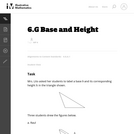Unrestricted Use
CC BY
Rating
0.0 stars

The purpose of this task is to help students understand what is meant by a base and its corresponding height in a triangle and to be able to correctly identify all three base-height pairs.

Subject:
Geometry
Mathematics
Material Type:
Activity/Lab
Provider:
Illustrative Mathematics
Provider Set:
Illustrative Mathematics
Author:
Illustrative Mathematics
05/01/2012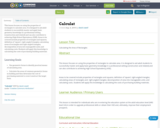Unrestricted Use
CC BY
Rating
0.0 stars

This lesson focuses on using the properties of rectangles to calculate area. It is designed to aid adult students to successfully master and apply basic geometry knowledge in a professional setting (construction and related) and can also contribute to achieving High School Equivalency (HSE).
Areas to be covered include properties of rectangles and squares; definition of ‘square’; right-angled triangles; calculating areas of rectangles and right-angled triangles; decomposition of area into manageable units; and calculating costs. Students will apply this knowledge to calculating the costs of purchasing building materials.

Subject:
Mathematics
Material Type:
Interactive
Lesson Plan
08/08/2019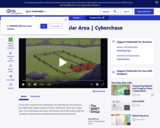Educational Use
Rating
0.0 stars

In this video segment from Cyberchase, the CyberSquad measures Judge Trudy's land claim by using tarps, fence posts and a grid made with rope.

Subject:
Mathematics
Material Type:
Lecture
Provider:
PBS LearningMedia
Provider Set:
PBS Learning Media: Multimedia Resources for the Classroom and Professional Development
Author:
U.S. Department of Education
WNET
07/07/2008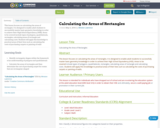Unrestricted Use
CC BY
Rating
0.0 stars

This lesson focuses on calculating the areas of rectangles. It is designed to enable adult students to successfully master basic geometry knowledge in order to achieve their High School Equivalency (HSE). Areas to be covered include types of polygons, quadrilaterals, rectangles; calculating areas of rectangle and calculating costs. Students will apply this knowledge to practical areas of their lives such as calculating the costs of purchasing carpets or painting of walls

Subject:
Mathematics
Material Type:
Interactive
Lesson Plan
05/05/2016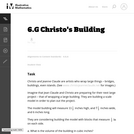Unrestricted Use
CC BY
Rating
0.0 stars

This task is primarily about volume and surface area, although it also gives students an early look at converting between measurements in scale models and the real objects they correspond to.

Subject:
Geometry
Mathematics
Material Type:
Activity/Lab
Provider:
Illustrative Mathematics
Provider Set:
Illustrative Mathematics
Author:
Illustrative Mathematics
05/01/2012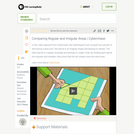Educational Use
Rating
0.0 stars

The CyberSquad proves that the area of Hacker's land is equal to the area of Judge Trudy's land in this video segment from Cyberchase.

Subject:
Mathematics
Material Type:
Lecture
Provider:
PBS LearningMedia
Provider Set:
PBS Learning Media: Multimedia Resources for the Classroom and Professional Development
Author:
U.S. Department of Education
WNET
07/08/2008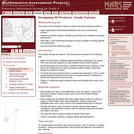Only Sharing Permitted
CC BY-NC-ND
Rating
0.0 stars

This lesson unit is intended to help teachers assess how well students are able to: Select appropriate mathematical methods to use for an unstructured problem; interpret a problem situation, identifying constraints and variables, and specify assumptions; work with 2- and 3-dimensional shapes to solve a problem involving capacity and surface area; and communicate their reasoning clearly.

Subject:
Geometry
Mathematics
Material Type:
Assessment
Lesson Plan
Provider:
Shell Center for Mathematical Education
Provider Set:
Mathematics Assessment Project (MAP)
04/26/2013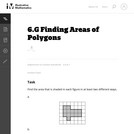Unrestricted Use
CC BY
Rating
0.0 stars

The purpose of this task is to have students work on a sequence of area problems that shows the advantage of increasingly abstract strategies in preparation for developing general area formulas for parallelograms and triangles.

Subject:
Geometry
Mathematics
Material Type:
Activity/Lab
Provider:
Illustrative Mathematics
Provider Set:
Illustrative Mathematics
Author:
Illustrative Mathematics
05/01/2012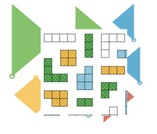Conditional Remix & Share Permitted
CC BY-NC
Rating
0.0 stars

Download GeoGebra Illustrative Mathematics LMS course files. All of GeoGebra's Illustrative Mathematics apps have been placed via links into Moodle courses - each unit is one course. There are twenty save middles school courses and more for high school (the list is growing.) These course files can then be uploaded into Moodle, Schoology, Canvas or D2L.

Subject:
Mathematics
Material Type:
Full Course
Author:
Dan MGuire
Diana Fisher Ph.D.
04/07/2021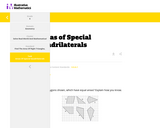Conditional Remix & Share Permitted
CC BY-NC-SA
Rating
0.0 stars

This task asks students to identify which of the six polygons have the same area. Students may complete the task using a variety of techniques including decomposing shapes, using transformations (rotations, reflections, translations) to move one or more parts of the figure to another part to more easily calculate the area, enclosing the polygon inside a larger rectangle and then subtract the areas of the "extra" pieces, etc.

Subject:
Mathematics
Material Type:
Activity/Lab
Diagram/Illustration
Lesson Plan
Provider:
Illustrative Mathematics
05/08/2020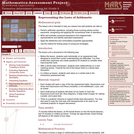Only Sharing Permitted
CC BY-NC-ND
Rating
0.0 stars

This lesson unit is intended to help you assess how well students are able to: Perform arithmetic operations, including those involving whole-number exponents, recognizing and applying the conventional order of operations; Write and evaluate numerical expressions from diagrammatic representations and be able to identify equivalent expressions; apply the distributive and commutative properties appropriately; and use the method for finding areas of compound rectangles.

Subject:
Geometry
Mathematics
Material Type:
Assessment
Lesson Plan
Provider:
Shell Center for Mathematical Education
Provider Set:
Mathematics Assessment Project (MAP)
04/26/2013Conditional Remix & Share Permitted
CC BY-NC
Rating
0.0 stars
Rating
0.0 stars
Subject:
Mathematics
Material Type:
Full Course
Provider:
Pearson
10/06/2016Conditional Remix & Share Permitted
CC BY-NC
Rating
0.0 stars

Surface Area and Volume

Type of Unit: Conceptual

Prior Knowledge

Students should be able to:

Identify rectangles, parallelograms, trapezoids, and triangles and their bases and heights.
Identify cubes, rectangular prisms, and pyramids and their faces, edges, and vertices.
Understand that area of a 2-D figure is a measure of the figure's surface and that it is measured in square units.
Understand volume of a 3-D figure is a measure of the space the figure occupies and is measured in cubic units.

Lesson Flow

The unit begins with an exploratory lesson about the volumes of containers. Then in Lessons 2–5, students investigate areas of 2-D figures. To find the area of a parallelogram, students consider how it can be rearranged to form a rectangle. To find the area of a trapezoid, students think about how two copies of the trapezoid can be put together to form a parallelogram. To find the area of a triangle, students consider how two copies of the triangle can be put together to form a parallelogram. By sketching and analyzing several parallelograms, trapezoids, and triangles, students develop area formulas for these figures. Students then find areas of composite figures by decomposing them into familiar figures. In the last lesson on area, students estimate the area of an irregular figure by overlaying it with a grid. In Lesson 6, the focus shifts to 3-D figures. Students build rectangular prisms from unit cubes and develop a formula for finding the volume of any rectangular prism. In Lesson 7, students analyze and create nets for prisms. In Lesson 8, students compare a cube to a square pyramid with the same base and height as the cube. They consider the number of faces, edges, and vertices, as well as the surface area and volume. In Lesson 9, students use their knowledge of volume, area, and linear measurements to solve a packing problem.

Subject:
Geometry
Mathematics
Material Type:
Unit of Study
Provider:
Pearson